# 1508 Hosting a Playful Math Carnival and Flying by the Seat of My Pants

### Blog Submission Appeal:

Please, tell me how you’ve made K-12 math education more fun. You see, later this month I’m hosting the Playful Math Education Blog Carnival. I have found several great blog posts to share, but maybe I haven’t seen yours. You can share your blog post with me by submitting this official form, leaving a comment below, or messaging me on twitter, Iva Sallay@findthefactors.com. I look forward to reading your post! Please share it with me by Saturday, August 22 so it can be included in this month’s carnival.

### Today’s Puzzle:

This mystery-level puzzle was modeled after a carnival ride, the swing carousel, a ride that tilts slightly as it goes around, and lets you ride by the seat of your pants. My puzzle might not be the best representation of that ride, but it hopefully got your attention.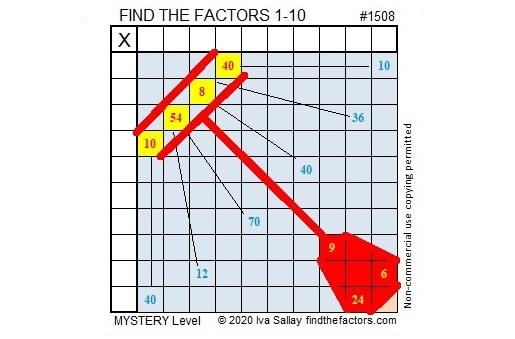Embellishing the puzzle might make it more eye-catching, but it is probably easier to solve the puzzle without distracting color and lines. (It’s a mystery-level puzzle, so I’m keeping how easy or difficult it is a secret.) Here is a plain version of the same puzzle: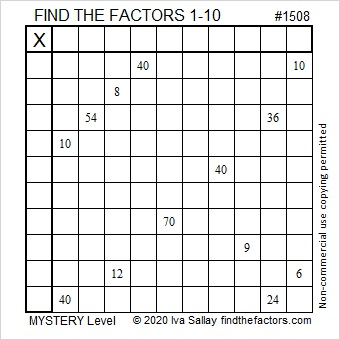### Factors of 1508:

• 1508 is a composite number.
• Prime factorization: 1508 = 2 × 2 × 13 × 29, which can be written 1508 = 2² × 13 × 29
• 1508 has at least one exponent greater than 1 in its prime factorization so √1508 can be simplified. Taking the factor pair from the factor pair table below with the largest square number factor, we get √1508 = (√4)(√377) = 2√377
• The exponents in the prime factorization are 2, 1, and 1. Adding one to each exponent and multiplying we get (2 + 1)(1 + 1)(1 + 1) = 3 × 2 × 2 = 12. Therefore 1508 has exactly 12 factors.
• The factors of 1508 are outlined with their factor pair partners in the graphic below.### More about the Number 1508:

1508 is the sum of two squares in two different ways:
32² + 22² = 1508
38² + 8² = 1508

1508 is the hypotenuse of FOUR Pythagorean triples:
540-1408-1508, calculated from 32² – 22², 2(32)(22), 32² + 22²,
580-1392-1508, which is (5-12-13) times 116,
608-1380-1508, calculated from 2(38)(8), 38² – 8², 38² + 8²,
1040-1092-1508 which is (20-21-29) times 52.

# 1507 and Level 6

### Today’s Puzzle:

Level six puzzles are designed to be tricky, but if you examine the clues, there is a logical place to begin, and logic can help you complete the entire puzzle.### Factors of 1507:

• 1507 is a composite number.
• Prime factorization: 1507 = 11 × 137
• 1507 has no exponents greater than 1 in its prime factorization, so √1507 cannot be simplified.
• The exponents in the prime factorization are 1, and 1. Adding one to each exponent and multiplying we get (1 + 1)(1 + 1) = 2 × 2 = 4. Therefore 1507 has exactly 4 factors.
• The factors of 1507 are outlined with their factor pair partners in the graphic below.### More about the Number 1507:

1507 is the hypotenuse of a Pythagorean triple.
968-1155-1507

A divisibility trick tells us that all of the numbers in that triple are divisible by 11:
9 – 6 + 8 = 11,
1 – 1 + 5 – 5 = 0,
1 – 5 + 0 – 7 = -11.

Yes, you would need to understand negative numbers for that last one, but 11, 0, and -11 can all be evenly divided by 11 so the corresponding numbers are also divisible by 11.

In fact, 968-1155-1507 is just 11 times (88-105-137).

# 1506 and Level 5

### Today’s Puzzle:

Can you find the factors 1 to 10 in a logical order so that the given clues are the products of those factors? Don’t let any of the clues trick you!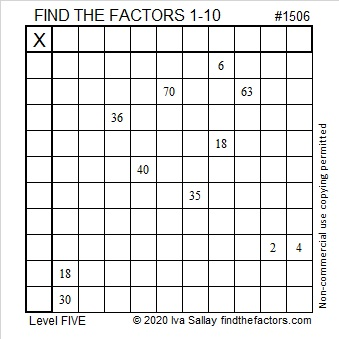### Factors of 1506:

• 1506 is a composite number.
• Prime factorization: 1506 = 2 × 3 × 251.
• 1506 has no exponents greater than 1 in its prime factorization, so √1506 cannot be simplified.
• The exponents in the prime factorization are 1, 1, and 1. Adding one to each exponent and multiplying we get (1 + 1)(1 + 1)(1 + 1) = 2 × 2 × 2 = 8. Therefore 1506 has exactly 8 factors.
• The factors of 1506 are outlined with their factor pair partners in the graphic below.### Pythagorean triples with 1506:

1506 is not the sum or the difference of two squares but it is still part of two Pythagorean triples:
1506-567008-567010, calculated from 2(753)(1), 753² – 1², 753² + 1², and
1506-62992-63010, calculated from 2(251)(3), 251² – 1², 251² + 1².

# 1505 and Level 4

### Today’s Puzzle:

If you’ve never done a level 4 puzzle before, this one is a great choice. It has fewer tricky clues than usual. Start with a row or column that has two clues. Use logic and multiplication facts to figure out where to put the factors 1 to 10 in both the first column and the top row so that the given clues are the products of those factors. Have fun!### Factors of 1505:

• 1505 is a composite number.
• Prime factorization: 1505 = 5 × 7 × 43.
• 1505 has no exponents greater than 1 in its prime factorization, so √1505 cannot be simplified.
• The exponents in the prime factorization are 1, 1, and 1. Adding one to each exponent and multiplying we get (1 + 1)(1 + 1)(1 + 1) = 2 × 2 × 2 = 8. Therefore 1505 has exactly 8 factors.
• The factors of 1505 are outlined with their factor pair partners in the graphic below.### More about the Number 1505:

1505 has four different factor pairs. The numbers in each factor pair add up to an even number, so 1505 is the difference of two squares in four different ways. Here’s the complete list of those ways:
753² – 752² = 1505
153² – 148² = 1505
111² – 104² = 1505
39² – 4² = 1505

# 1504 and Level 3

### Today’s Puzzle:

Since this is a level 3 puzzle the clues are given in a logical order from top to bottom. Write the factors 1 to 10 in the first column and again in the top row.

Usually, you only have to consider the previous clues when finding the factors in a level 3 puzzle, but when you consider if 4 = 2 × 2 or 1 × 4, you will also have to look at a clue below it. You can do this!### Factors of 1504:

• 1504 is a composite number.
• Prime factorization: 1504 = 2 × 2 × 2 × 2 × 2 × 47, which can be written 1504 = 2⁵ × 47
• 1504 has at least one exponent greater than 1 in its prime factorization so √1504 can be simplified. Taking the factor pair from the factor pair table below with the largest square number factor, we get √1504 = (√16)(√94) = 4√94
• The exponents in the prime factorization are 5 and 1. Adding one to each exponent and multiplying we get (5 + 1)(1 + 1) = 6 × 2 = 12. Therefore 1504 has exactly 12 factors.
• The factors of 1504 are outlined with their factor pair partners in the graphic below.### More about the Number 1504:

1504 is the difference of two squares in four different ways:
377² – 375² = 1504
190² – 186² = 1504
98² – 90² = 1504
55² – 39² = 1504

# Why is 1503 a Friedman Number?

### Friedman Puzzle:

Can you find an expression equaling 1503 that uses 1, 5, 0, and 3 each exactly once, but in any order, and some combination of  +, -, ×, or ÷? For this particular Friedman puzzle, none of those digits are exponents. If you can solve this Friedman puzzle, you will know why 1503 is the 24th Friedman number. You can find the solution hidden someplace in this post. (By the way, another permutation of those digits, 1530, will be the 25th Friedman number!)

### Find the Factors Puzzle:

There are 14 clues in this level 2 puzzle. Use those clues and logic to place the factors 1 to 10 in both the first column and the top row. That’s how you start to turn this puzzle into a multiplication table!### Factors of 1503:

• 1503 is a composite number.
• Prime factorization: 1503 = 3 × 3 × 167, which can be written 1503 = 3² × 167
• 1503 has at least one exponent greater than 1 in its prime factorization so √1503 can be simplified. Taking the factor pair from the factor pair table below with the largest square number factor, we get √1503 = (√9)(√167) = 3√167
• The exponents in the prime factorization are 2 and 1. Adding one to each exponent and multiplying we get (2 + 1)(1 + 1) = 3 × 2 = 6. Therefore 1503 has exactly 6 factors.
• The factors of 1503 are outlined with their factor pair partners in the graphic below.Did you see the solution to the Friedman puzzle in that factor pair chart?

# 1502 and Level 1

### Today’s Puzzle:

This level 1 puzzle has products in one of the rows and in one of the columns. Can you use those products to figure out where the factors 1 to 10 belong in this multiplication table puzzle?### Factors of 1502:

• 1502 is a composite number.
• Prime factorization: 1502 = 2 × 751
• 1502 has no exponents greater than 1 in its prime factorization, so √1502 cannot be simplified.
• The exponents in the prime factorization are 1, and 1. Adding one to each exponent and multiplying we get (1 + 1)(1 + 1) = 2 × 2 = 4. Therefore 1502 has exactly 4 factors.
• The factors of 1502 are outlined with their factor pair partners in the graphic below.### Pythagorean Triple with 1502:

1502 is not the sum or the difference of two squares, but 1502 = 2(751)(1), so it is part of a Pythagorean triple:
1502-564000-564002, calculated from 2(751)(1), 751² – 1², 751² – 1².

# 1501 is a Centered Pentagonal Number

### 1501 Tiny Dot Puzzle

A long time ago before calculators or computers existed, someone determined that 1501 tiny dots could be formed into a pentagon. That was a remarkable puzzle to complete! What kind of pentagon will 1501 tiny dots make?

Since 5(24)(25)/2 + 1 = 1501, it is the 25th centered pentagonal number.  Notice in the graphic below that those 1501 tiny dots can also be divided into 5 equally-sized triangles with just the center dot leftover.

### Factors of 1501:

• 1501 is a composite number.
• Prime factorization: 1501 = 19 × 79
• 1501 has no exponents greater than 1 in its prime factorization, so √1501 cannot be simplified.
• The exponents in the prime factorization are 1, and 1. Adding one to each exponent and multiplying we get (1 + 1)(1 + 1) = 2 × 2 = 4. Therefore 1501 has exactly 4 factors.
• The factors of 1501 are outlined with their factor pair partners in the graphic below.### Another fact about the number 1501:

1501 is the difference of two squares in two different ways:
751² – 750² = 1501,
49² – 30² = 1501.

# Celebrating 1500 with a Horse Race and Much More!

Writing 1500 posts is quite a milestone. I’ll begin the celebration with an exciting horse race! Let me explain:

Each prime number has exactly 2 factors. Every composite number between 1401 and 1500 has somewhere between 4 and 36 factors. Which quantity of factors do you think will appear most often for these numbers? Pick that amount as your pony and see how far it gets in this horse race!make science GIFs like this at MakeaGif

Did the race results surprise you? They surprised me!

### Prime Factorization for numbers from 1401 to 1500:

Here’s a chart showing the prime factorization of all those numbers and the amount of factors each number has. Numbers in pink have exponents in their prime factorization so their square roots can be simplified: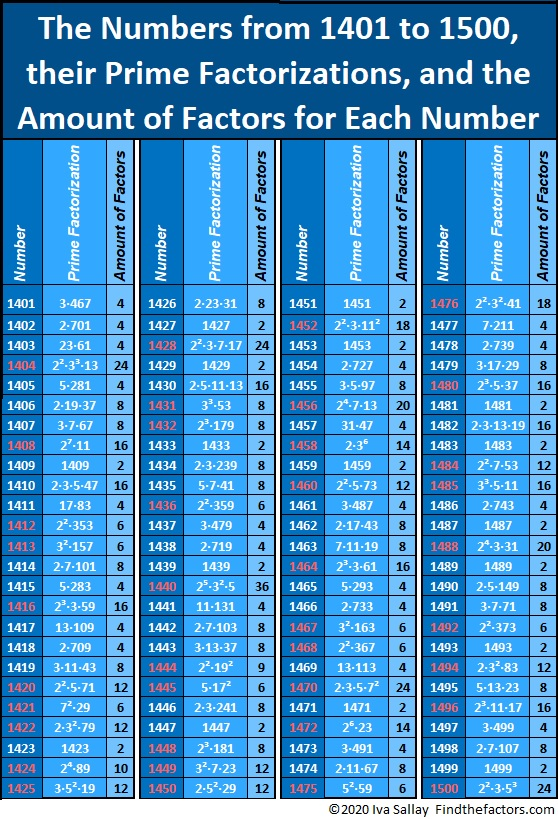### Today’s Puzzles:

Let’s continue the celebration with a puzzle: 1500 has 12 different factor pairs. One of those pairs adds up to 85 and one of them subtracts to give 85. Can you find those factor pairs that make sum-difference and write them in the puzzle? You can look at all of the factor pairs of 1500 in the graphic after the puzzle, but the second puzzle is really just the first puzzle in disguise. So try solving that easier puzzle first.### Factors of 1500:

• 1500 is a composite number.
• Prime factorization: 1500 = 2 × 2 × 3 × 5 × 5 × 5, which can be written 1500 = 2² × 3 × 5³
• 1500 has at least one exponent greater than 1 in its prime factorization so √1500 can be simplified. Taking the factor pair from the factor pair table below with the largest square number factor, we get √1500 = (√100)(√15) = 10√15
• The exponents in the prime factorization are 2, 1, and 3. Adding one to each exponent and multiplying we get (2 + 1)(1 + 1)(3 + 1) = 3 × 2 × 4 = 24. Therefore 1500 has exactly 24 factors.
• The factors of 1500 are outlined with their factor pair partners in the graphic below.### A Forest of Factor Trees:

Take a few minutes to hike in this forest featuring a few of the MANY possible factor trees for 1500. Celebrate each tree’s uniqueness!### Other Facts to Celebrate about 1500:

Oeis.org tells us that  (5+1) × (5+5) × (5+0) × (5+0) = 1500.

1500 is the hypotenuse of THREE Pythagorean triples:
420-1440-1500, which is (7-24-25) times 60,
528-1404-1500, which is 12 times (44-117-225),
900-1200-1500, which is (3-4-5) times 300.

# 1498 Another Mystery

### Today’s Puzzle:

The number 48 appears four times in a 12 × 12 multiplication table, and all four 48’s appear in this puzzle! Where will you put its factors, 6, 8, 4, 12? Use logic to figure out where all the numbers from 1 to 12 need to go to make this puzzle turn into a multiplication table.### Factors of 1498:

• 1498 is a composite number.
• Prime factorization: 1498 = 2 × 7 × 107.
• 1498 has no exponents greater than 1 in its prime factorization, so √1498 cannot be simplified.
• The exponents in the prime factorization are 1, 1, and 1. Adding one to each exponent and multiplying we get (1 + 1)(1 + 1)(1 + 1) = 2 × 2 × 2 = 8. Therefore 1498 has exactly 8 factors.
• The factors of 1498 are outlined with their factor pair partners in the graphic below.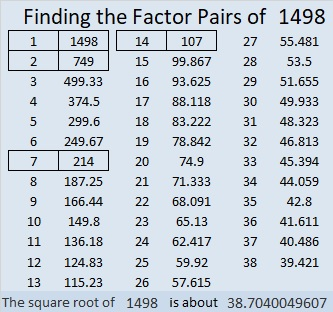### Pythagorean Triples with 1498:

1498 is 2(107)(7) so we can calculate a cool Pythagorean triple from
2(107)(7), 107² – 7², 107² + 7² to get 1498-11400-11498.

Also from 2(749)(1), 749² – 1², 749² + 1², we get 1498-561000-561002.